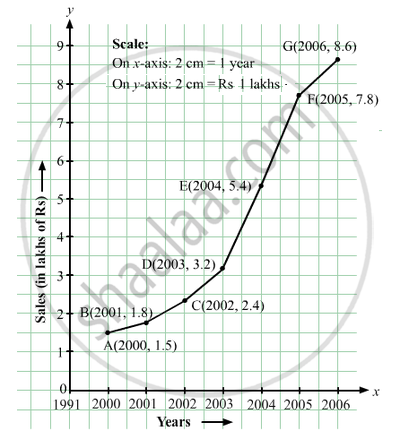Advertisement Remove all ads

# The Following Table Shows the Sales of a Commodity During the Years 2000 to 2006. - Mathematics

Answer in Brief

The following table shows the sales of a commodity during the years 2000 to 2006.

 Years: 2000 2001 2002 2003 2004 2005 2006 Sales (in lakhs of Rs): 1.5 1.8 2.4 3.2 5.4 7.8 8.6

Draw a graph of this information.

Advertisement Remove all ads

#### Solution

Here, year is an independent variable and sales is a dependent variable. So, we take year on the x-axis and sales on the y-axis.
Let us choose the following scale:
On x-axis: 2 cm = 1 year
On y-axis: 2 cm = 1 lakh rupees
Assume that on x-axis, origin (O) represents 1991.
So, the coordinates of O are (1991,0).
Now, let us plot (2000, 1.5), (2001, 1.8), (2002, 2.4), (2003, 3.2), (2004, 5.4), (2005, 7.8) and (2006, 8.6). These points are joined to get the graph representing the given information as shown in the figure below.​Is there an error in this question or solution?
Advertisement Remove all ads

#### APPEARS IN

RD Sharma Class 8 Maths
Chapter 27 Introduction to Graphs
Exercise 27.2 | Q 5 | Page 16
Advertisement Remove all ads
Advertisement Remove all ads
Share
Notifications

View all notifications

Forgot password?
Course